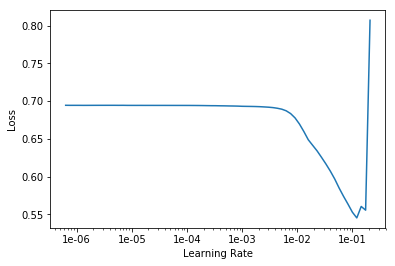# IoU metrics Segmentation

I am trying to use IoU metrics in a segmentation problem. I just copied the metrics code and pasted into my notebook:

``````def dice(input:Tensor, targs:Tensor, iou:bool=True)->Rank0Tensor:
"Dice coefficient metric for binary target. If iou=True, returns iou metric, classic for segmentation problems."
n = targs.shape
input = input.argmax(dim=1).view(n,-1)
targs = targs.view(n,-1)
intersect = (input*targs).sum().float()
union = (input+targs).sum().float()
if not iou: return 2. * intersect / union
else: return intersect / (union-intersect+1.0)
``````

I have changed iou=True to get the IoU metrics and passed this as metrics:

``````metrics = dice
learn = Learner.create_unet(data, models.resnet34, metrics=metrics)

lr_find(learn)
learn.recorder.plot()
``````

The plot is weird and I tried different learning rates (1e-2, 1e-02, etc)Now, My validation numbers and metrics are all zeros. What am I doing wrong?

``````epoch  train_loss  valid_loss  dice
1      0.138745    nan         0.000000  (01:17)
2      0.134525    nan         0.000000  (01:15)``````
2 Likes

Metric shouldn’t matter. In plot you have loss which is different. Also when we look your valid_loss I would say that there is some problem with your data or how you implement it. Can you tell us something about your data.

2 Likes

these both are the same

1 Like

@shakur any idea why your valid_loss is too high?

1 Like

I am still strugling to find out why?